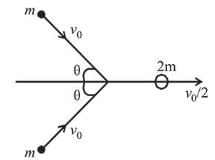# Two bodies of the same mass are moving with the same speed,

Question:

Two bodies of the same mass are moving with the same speed, but in different directions in a plane. They have a completely inelastic collision and move together thereafter with a final speed which is half of their initial speed. The angle between the initial velocities of the two bodies (in degree) is______Solution:Momentum conservation along $x$ direction,

$2 m v_{0} \cos \theta=2 m \frac{v_{0}}{2} \Rightarrow \cos \theta=\frac{1}{2}$ or $\theta=60^{\circ}$

Hence angle between the initial velocities of the two bodies

$=\theta+\theta=60^{\circ}+60^{\circ}=120^{\circ}$